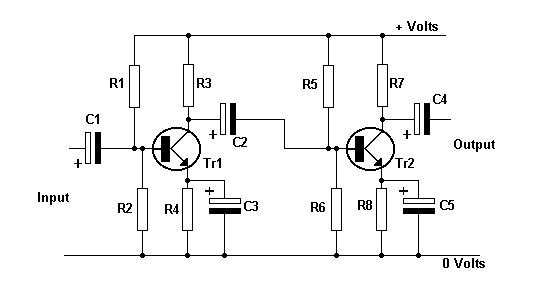Home > Electronic Tutorials > Junction Transistors Tutorial > Amplifiers in Cascade Tutorial

Junction Transistors Electronic TutorialsIf the gain of one stage of amplification is insufficient, then two or more stages can be connected in cascade, as shown.

If the gain of each stage is 50 times, then the overall gain is 50 x 50 =2,500.

If the input to Tr1 is 1 mV, then the output of Tr2 is 2,500 mV =2.5Volts.

If the gain is excessive the circuit may be unstable, or the output may be clipped.